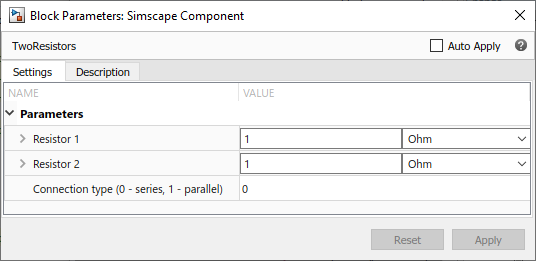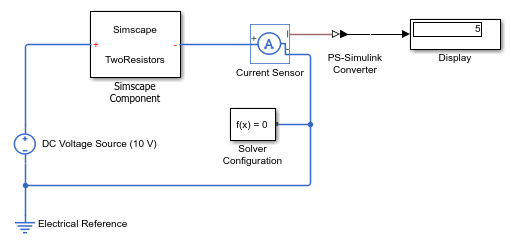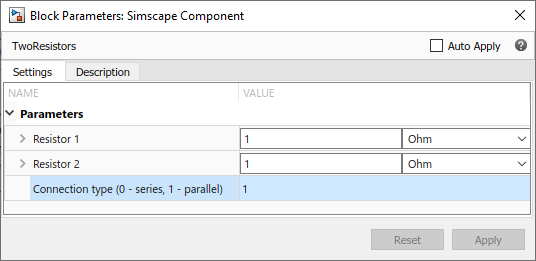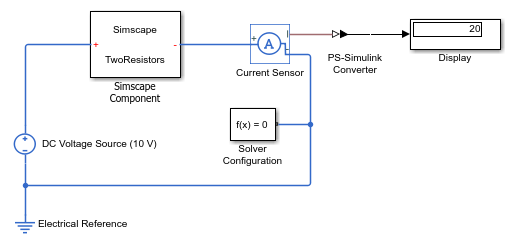## Defining Component Variants

Physical modeling often requires incremental modeling approach. It is a good practice to start with a simple model, run and troubleshoot it, then add the desired special effects, like fluid compressibility or fluid inertia. Another example is modeling a diode with different levels of complexity: linear, zener diode, or exponential. Composite components often require conditional inclusion of a certain member component and a flexible connection scheme.

Including different modeling variants within a single component requires applying control logic to determine the model configuration. You achieve this goal by using conditional sections in a component file.

### Conditional Sections

A conditional section is a top-level section guarded by an `if` clause. Conditional sections are parallel to other top-level sections of a component file, such as declaration or equations sections.

A conditional section starts with an `if` keyword and ends with an `end` keyword. It can have optional `elseif` and `else` branches. The body of each branch of a conditional section can contain declaration blocks, equations, structure sections, and so on, but cannot contain the `setup` function.

The `if` and `elseif` branches start with a predicate expression. If a predicate is true, the branch gets activated. When all predicates are false, the `else` branch (if present) gets activated. The compiled model includes elements (such as declarations, equations, and so on) from active branches only.

```component MyComp [...] if Predicate1 [...] % body of branch1 elseif Predicate2 [...] % body of branch2 else [...] % body of branch3 end [...] end ```

Unlike the `if` statements in the equations section, different branches of a conditional section can have different variables, different number of equations, and so on. For example, you can have two variants of a pipe, one that accounts for resistive properties only and the second that also models fluid compressibility:

```component MyPipe parameters fl_c = 0; % Model compressibility? (0 - no, 1 - yes) end [...] % other parameters, variables, branches if fl_c == 0 equations % first set of equations, resistive properties only end else variables % additional variable declarations, needed to account for fluid compressibility end equations % second set of equations, including fluid compressibility end end end ```

In this example, if the block parameter Model compressibility? (0 - no, 1 - yes) is set to 0, the first set of equations gets activated and the block models only the resistive properties of the pipe. If the block user changes the value of the parameter, then the `else` branch gets activated, and the compiled model includes the additional variables and equations that account for fluid compressibility.

Note

Enumerations are very useful in defining component variants, because they let you specify a discrete set of acceptable parameter values. For an example of how this component can use enumeration, see Using Enumeration in Predicates.

### Rules and Restrictions

Nested conditional sections are allowed. For example:

```component A parameters p1 = 0; p2 = 0; p3 = 0; end if p1 > 0 [...] if p2 > 0 [...] end if p3 > 0 [...] end [...] end end ```

Predicates must be parametric expressions, because the structure of a model must be fixed at compile time and cannot change once the model is compiled. Using a variable in a predicate results in a compile-time error:

```component A [...] variables v = 0; end if v > 0 % error: v>0 is not a parametric expression because v is a variable [...] else [...] end end ```

Predicates may depend on parameters of the parent (enclosing) component. They may not depend, directly or indirectly, on parameters of member (embedded) components or on domain parameters:

```component A parameters p = 1; end parameters(Access=private) pp = c.p; end components c = MyComp; end nodes n = MyDomain; end if p > 0 % ok [...] elseif c.p > 0 % error: may not depend on parameters of embedded component [...] elseif n.p > 0 % error: may not depend on domain parameters [...] elseif pp > 0 % error: pp depends on c.p [...] end end ```

Accessibility of class members declared inside conditional sections is equivalent to private class members `(Access=private)`. They are not accessible from outside the component class, even if their branch is active.

The scope of the class members declared inside a conditional section is the entire component class. For example:

```component A nodes p = foundation.electrical.electrical; n = foundation.electrical.electrical; end parameters p1 = 1; end if p1 > 0 components r1 = MyComponentVariant1; end else components r1 = MyComponentVariant2; end end connections connect(p, r1.p); connect(n, r1.n); end end ```

However, using a conditional member outside the conditional section when the branch is not active results in a compilation error:

```component A nodes p = foundation.electrical.electrical; n = foundation.electrical.electrical; end parameters p1 = 0; end if p1 > 0 components r1 = MyComponentVariant1; end end connections connect(p, r1.p); % error if p1=0 and the predicate is false end end ```

Parameters that are referenced by predicates of conditional sections, directly and indirectly, must be compile-time parameters. The `setup` function may not write to these parameters, for example:

```component A parameters p1 = 1; end if p1 > 0 % p1 is a compile-time parameter [...] else [...] end function setup tmp = p1; % ok to read from p1 p1 = 10; % error: may not write to p1 here end end ```

### Example

This simple example shows a component containing two resistors. The resistors can be connected either in series or in parallel, depending on the value of the control parameter:

```component TwoResistors nodes p = foundation.electrical.electrical; % +:left n = foundation.electrical.electrical; % -:right end parameters p1 = {1, 'Ohm'}; % Resistor 1 p2 = {1, 'Ohm'}; % Resistor 2 ct = 0; % Connection type (0 - series, 1 - parallel) end components(ExternalAccess=observe) r1 = foundation.electrical.elements.resistor(R=p1); r2 = foundation.electrical.elements.resistor(R=p2); end if ct == 0 % linear connection connections connect(p, r1.p); connect(r1.n, r2.p); connect(r2.n, n); end else % parallel connection connections connect(r1.p, r2.p, p); connect(r1.n, r2.n, n); end end end ```

To test the correct behavior of the conditional section, point a Simscape Component block to this component file. Place the block in a circuit with a 10V DC voltage source and a current sensor. With the default parameter values, the resistors are connected in series, and the current is 5A.If you change the value of the Connection type (0 - series, 1 - parallel) parameter to `1`, the resistors are connected in parallel, and the current is 20A.